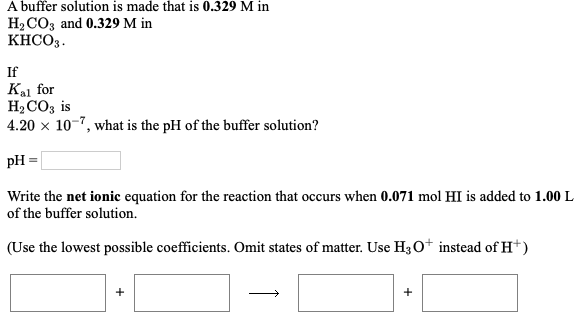# A buffer solution is made that is 0.329 M in H2CO3 and 0.329 M in KHCO3. I Ka1 for H2CO3 is 4.20x10^-7, what is the pH of the buffer solution? Write the net ionic equation for the reaction that occurs when 0.071 mol HI is added to 1.0 L of the buffer solution. (Use the lowest possible coefficients. Omit states of mater. Use H#O^+ instead of H^+)